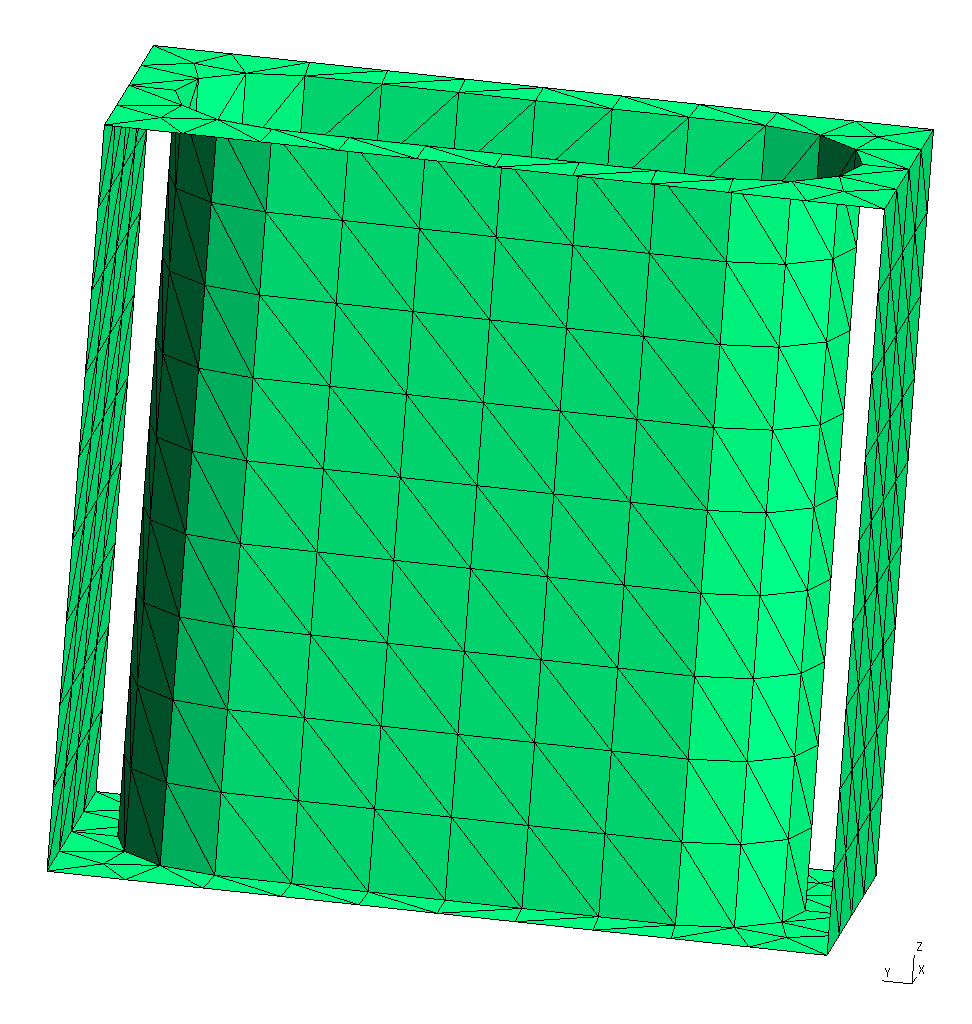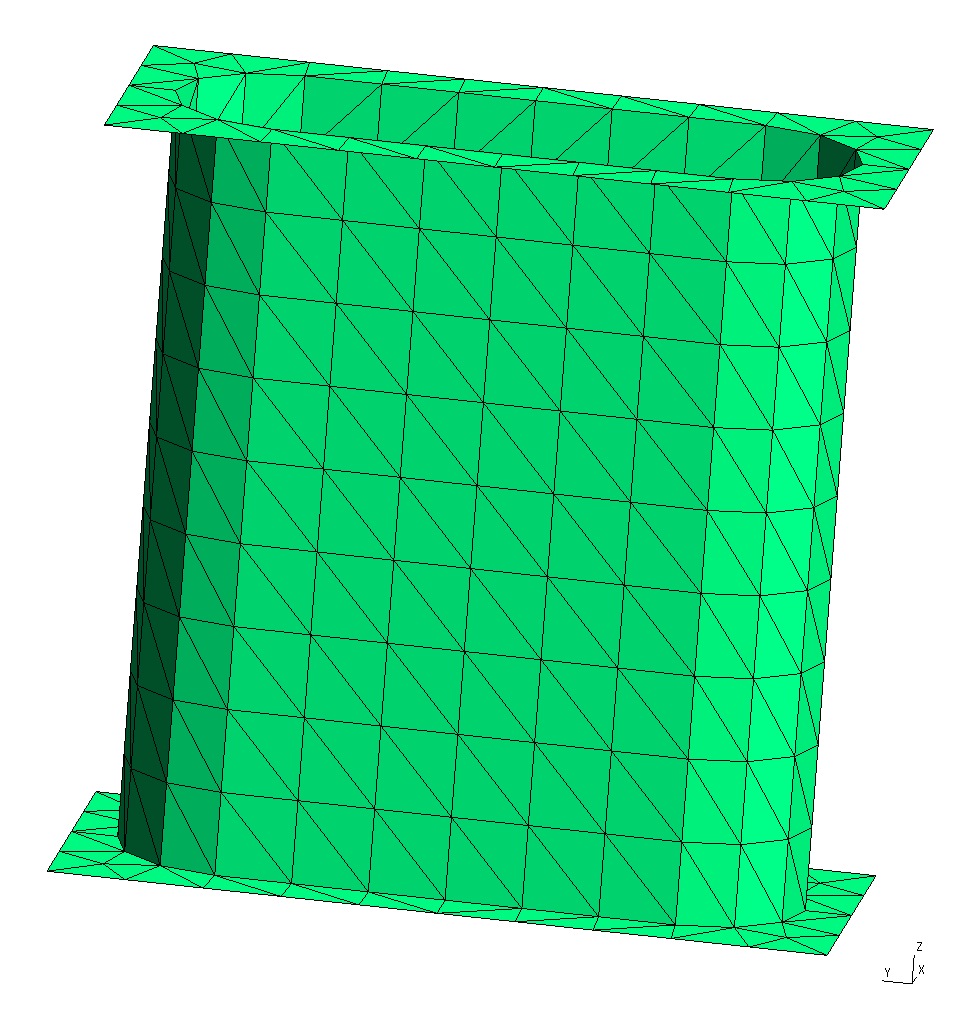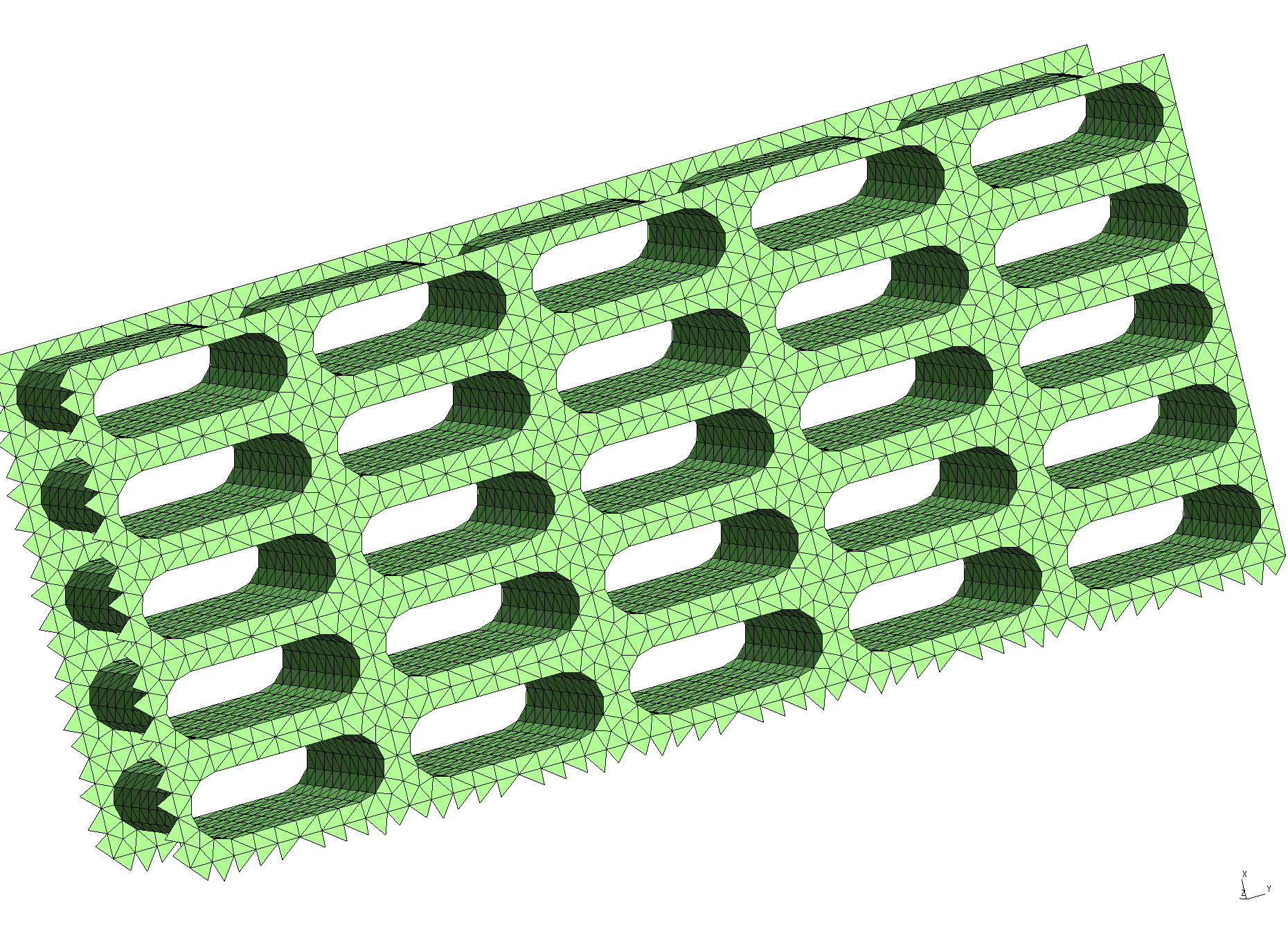Casimir-Polder potentials in dielectric nanostructures

In this example, we exploit scuff-em's support for periodic geometries to compute Casimir-Polder potentials for atoms inside dielectric waveguides. Our basic test example will be the 1D photonic crystal studied in this paper:

We will illustrate the use of scuff-em's Casimir-Polder module scuff-caspol by reproducing the results of Hung et al. for a 1D lattice, then extend the calculation to the case of a 2D square lattice. The files for this example may be found in the share/scuff-em/examples/NanobeamCasimirPolder subdirectory of your scuff-em installation.

gmsh geometry and surface mesh for nanobeam unit cell

The gmsh geometry file UnitCell.geo describes just the portion of the nanobeam surface that lies within the unit cell, i.e. the cell that is infinitely periodically replicated to yield the full geometry. To produce a discretized surface-mesh representation of this geometry, we run it through gmsh:

% gmsh -2 UnitCell.geo

This produces a file named UnitCell.msh, which I rename to NanoBeamUnitCell_1006_.msh because (a) I will be using it as the unit cell of a beam geometry, in contrast to a different use I will find for the same unit cell below; and (b) the mesh has 1006 interior edges (this is the number that defines the memory and computation time requirements for the scuff-em calculation; it may be found by running scuff-analyze --mesh UnitCell.msh). You can open the .msh file in gmsh to visualize the unit-cell mesh:

% gmsh NanoBeamUnitCell_1006.mshNote the following:

• For 1D periodic geometries in scuff-em, the direction of infinite extent must be the x direction.

• The sidewalls normal to the $y$ and $z$ directions are meshed, but the sidewalls normal to the $x$ direction are not meshed for this structure, because those surfaces are not interfaces between different dielectrics.

• For surfaces that straddle the unit-cell boundaries (as is the case here), each triangle edge that lies on the unit-cell boundary must have an identical image edge on the opposite side of the unit cell. An easy way to achieve this is to use extrusions in gmsh, as in the .geo file above.

• In this case the unit cell is 0.367 $\mu$m long. This and other geometric parameters can be modified by editing the file UnitCell.geo or directly on the gmsh commmand line using the -setnumber option.

scuff-em geometry file for dielectric nanobeam

A scuff-em [geometry file]scuffEMGeometries describing an extended nanobeam consisting of infinitely many repetitions of the above unit cell filled with a dielectric material of constant relative permittivity $\epsilon=4$, is NanoBeam_1006.scuffgeo. The file reads, in its entirety,

LATTICE
VECTOR 0.367 0.0
ENDLATTICE

OBJECT Nanobeam
MESHFILE NanoBeamUnitCell_1006.msh
MATERIAL CONST_EPS_4
ENDOBJECT

We can use scuff-analyze to visualize the geometry described by this .scuffgeo file:

% scuff-analyze --geometry NanoBeam_1006.scuffgeo --WriteGMSHFiles --Neighbors 3

[The option --Neighbors 3 requests that, in addition to the unit-cell geometry, the first 3 periodic images of the unit cell in both the positive and negative directions (for a total of 5 copies of the unit cell) be plotted as well. This helps to convey a slightly better sense of the actual infinite-length structure being simulated.] This produces the file NanoBeam_1006.pp, which you can view in gmsh:

% gmsh NanoBeam_1006.ppNote that the visualization file produced by scuff-analyze includes extra triangles (visible at the left end of the structure) that are not present in the unit-cell geometry. These are called straddlers, and they are added automatically by scuff-em to account for surface currents that flow across the unit-cell boundaries in periodic geometries.

gmsh geometry and surface mesh for nanoarray unit cell

It's easy to generalize all of this to a geometry with two-dimensional periodicity. The only modification required to the unit-cell mesh is that we have to remove the sidewalls normal to the $y$ direction. This can be done using the same gmsh geometry we used above for the nanobeam unit cell, but with the extra command-line option -setnumber LDim 2 on the gmsh command line. (Here LDim stands for "lattice dimension".)

% gmsh -2 -setnumber LDim 2 UnitCell.geo

This produces a file named UnitCell.msh, which I rename to NanoArrayUnitCell_800_.msh. It looks like thisscuff-em geometry file for dielectric nanoarray

A scuff-em geometry file describing a dielectric surface extended in two dimensions and perforated with a square lattice of the holes pictured above is NanoArray_800.scuffgeo. The file reads

LATTICE
VECTOR 0.367  0.0
VECTOR 0.000  0.845
ENDLATTICE

OBJECT NanoArray
MESHFILE NanoArrayUnitCell_800.msh
MATERIAL CONST_EPS_4
ENDOBJECT

Again we use scuff-analyze to visualize the geometry described by this .scuffgeo file:

% scuff-analyze --geometry NanoArray_800.scuffgeo --WriteGMSHFiles --Neighbors 3Evaluation points for Casimir-Polder potentials

For a Casimir-Polder calculation we will want to evaluate the Casimir-Polder potential at range of points. We put the Cartesian coordinates of these points into a text file named EPFile, which looks like this:

0.1835 0.4225 -0.500
0.1835 0.4225 -0.480
0.1835 0.4225 -0.460
...
0.1835 0.4225 0.480
0.1835 0.4225 0.500

and defines a line of points running through the middle of the hole in the beam unit cell.

To double-check that the evaluation points we specify are actually where we expect them to be vis-a-vis the meshed surfaces in our problem, we can ask scuff-analyze to plot the evaluation points together with the unit-cell geometry:

% scuff-analyze --geometry NanoBeam_1006.scuffgeo --EPFile EPFile --WriteGMSHFilesThis confirms that our EPFile describes a line of evaluation points running through the middle of the hole in the beam structure, as desired.

Setting up the Casimir-Polder calculation

To compute the Casimir-Polder potential on, say, a rubidium atom at the above evaluation points for the 1D and 2D extended structures, we now say simply

% scuff-caspol --geometry NanoBeam_1006.scuffgeo --EPFile EPFile --Atom Rubidium

and/or

% scuff-caspol --geometry NanoArray_800.scuffgeo --EPFile EPFile --Atom Rubidium

These calculations will produce files named NanoBeam_1006.out and NanoArray_800.out tabulating the Casimir-Polder potential experience by the rubidium atom at each of the specified evaluation points.

For more information on scuff-caspol, see the old scuff-caspol documentation, which is thorough and up-to-date (though it does not cover CP calculations in extended geometries) despite having not yet been ported from its previous format.